2022 NL MVP Odds
+350
3.5 to 1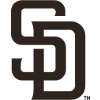Manny Machado
14.8% implied probability

+500
5 to 1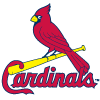Nolan Arenado
11.1% implied probability

+800
8 to 1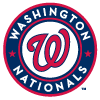Juan Soto
7.4% implied probability

+1000
10 to 1Bryce Harper
6.1% implied probability

+1100
11 to 1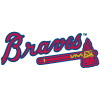Ronald Acuna
5.6% implied probability

+1200
12 to 1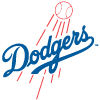Freddie Freeman
5.1% implied probability

+1500
15 to 1Mookie Betts
4.2% implied probability

+1800
18 to 1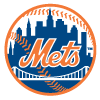Pete Alonso
3.5% implied probability

+2000
20 to 1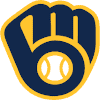Christian Yelich
3.2% implied probability

+2500
25 to 1Trea Turner
2.6% implied probability

+2500
25 to 1Francisco Lindor
2.6% implied probability

+3000
30 to 1Matt Olson
2.2% implied probability

+3000
30 to 1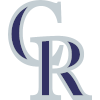C.J. Cron
2.2% implied probability

+4000
40 to 1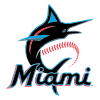Jazz Chisholm
1.6% implied probability

+5000
50 to 1Paul Goldschmidt
1.3% implied probability

+5000
50 to 1Austin Riley
1.3% implied probability

+5000
50 to 1Willy Adames
1.3% implied probability

+5000
50 to 1Ozzie Albies
1.3% implied probability

+5000
50 to 1Nick Castellanos
1.3% implied probability

+5000
50 to 1Fernando Tatis
1.3% implied probability

+8000
80 to 1Marcell Ozuna
0.8% implied probability

+8000
80 to 1Starling Marte
0.8% implied probability

+8000
80 to 1Kris Bryant
0.8% implied probability

+8000
80 to 1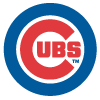Seiya Suzuki
0.8% implied probability

+8000
80 to 1Cody Bellinger
0.8% implied probability

+8000
80 to 1Max Muncy
0.8% implied probability

+10000
100 to 1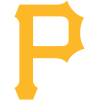Bryan Reynolds
0.7% implied probability

+10000
100 to 1Corbin Burnes
0.7% implied probability

+10000
100 to 1Jake Cronenworth
0.7% implied probability

+10000
100 to 1Jeff McNeil
0.7% implied probability

+10000
100 to 1Walker Buehler
0.7% implied probability

+10000
100 to 1Kyle Schwarber
0.7% implied probability

+10000
100 to 1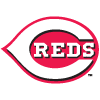Joey Votto
0.7% implied probability

+10000
100 to 1Tyler O'Neill
0.7% implied probability

+10000
100 to 1Rhys Hoskins
0.7% implied probability

+10000
100 to 1J.T. Realmuto
0.7% implied probability

+10000
100 to 1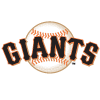Brandon Crawford
0.7% implied probability

+10000
100 to 1Brandon Belt
0.7% implied probability

+10000
100 to 1Dylan Carlson
0.7% implied probability

+10000
100 to 1Max Scherzer
0.7% implied probability

+12500
125 to 1Trent Grisham
0.5% implied probability

+12500
125 to 1Nelson Cruz
0.5% implied probability

+12500
125 to 1Justin Turner
0.5% implied probability

+12500
125 to 1Jonathan India
0.5% implied probability

+12500
125 to 1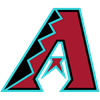Ketel Marte
0.5% implied probability

+15000
150 to 1Josh Bell
0.4% implied probability

+20000
200 to 1Eric Hosmer
0.3% implied probability

+20000
200 to 1Frank Schwindel
0.3% implied probability

+20000
200 to 1Ryan McMahon
0.3% implied probability

+20000
200 to 1Ke'Bryan Hayes
0.3% implied probability

+20000
200 to 1Willson Contreras
0.3% implied probability

+20000
200 to 1Jacob deGrom
0.3% implied probability

+30000
300 to 1Avisail Garcia
0.2% implied probability

+30000
300 to 1Jean Segura
0.2% implied probability

+30000
300 to 1Charlie Blackmon
0.2% implied probability

+50000
500 to 1Paul DeJong
0.1% implied probability

+50000
500 to 1Mike Moustakas
0.1% implied probability